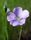## Assistance with a Count + Amount from a large table.

Hello there. I'm after some more help if I may.

I've been asked to extract some numbers from a partularly large table. I would normally use a data step but the table is massive.

The columns I would use would be

ID / Date / Amount / IntCode / Currency

I need to summarise 12 months worth of data and to extract it would take some time I believe.

is there a Proc Summary procedure whereby I could say:-

If the date is in 2012 and the Currency is 978 then show me the Count of records and the sumed value of the amount column?

I'm not great with Proc procedures so appologies in advance for the basic question.

Kind Regards

Stret

1 ACCEPTED SOLUTION

Accepted Solutions

## Re: Assitance with a Count + Amount from a large table.

Basic tools that you'll need to know eventually ... here's a shell of a program.

proc means data=have sum n nmiss;

var amount;

where /* something here to subset records */;

run;

The WHERE statement depends on what your data actually contains.  Is CURRENCY character or numeric?  Is DATE a SAS date value or a datetime value?  For one set of answers, this would match:

where currency='978' and year(date) = 2012;

However, the fastest solution might conceivably be a DATA step, if there is a known order to the observations.  For example, if the records are sorted by DATE, a DATA step could use:

if year(date) > 2012 then do;

* print results;

stop;

run;

Then there would be no need to examine the remaining records.  (The WHERE statement has to examine all records even if it doesn't use them in the computations.)

6 REPLIES 6

## Re: Assistance with a Count + Amount from a large table.

Hi, maybe something like this?

``````proc summary data=massive
(where=(year(date)=2012 and currency=978));
var amount;
output out=result n=N sum=Sum;
run;
``````

Assuming Currency is some sort of code, not a variable you need to summarize.

## Re: Assitance with a Count + Amount from a large table.

Basic tools that you'll need to know eventually ... here's a shell of a program.

proc means data=have sum n nmiss;

var amount;

where /* something here to subset records */;

run;

The WHERE statement depends on what your data actually contains.  Is CURRENCY character or numeric?  Is DATE a SAS date value or a datetime value?  For one set of answers, this would match:

where currency='978' and year(date) = 2012;

However, the fastest solution might conceivably be a DATA step, if there is a known order to the observations.  For example, if the records are sorted by DATE, a DATA step could use:

if year(date) > 2012 then do;

* print results;

stop;

run;

Then there would be no need to examine the remaining records.  (The WHERE statement has to examine all records even if it doesn't use them in the computations.)

## Re: Assitance with a Count + Amount from a large table.

Thank you for the swift responses, they both make complete sense.

These two will sum the Amount variable correct?

If i wanted to show the count of obervations from the same criteria could I do that in a similar way?

Again thank you for the help

Stret

## Re: Assitance with a Count + Amount from a large table.

Both will sum AMOUNT.

The primary difference:  PROC SUMMARY does not print its result by default.  So the OUTPUT statement creates a SAS data set holding the summary.  PROC MEANS prints its results by default.  You can add an OUTPUT statement to it, but that's not needed if all  you want is a printed report.  PROC SUMMARY and PROC MEANS have the exact same capabilities, but with different defaults.

The output data set will contain an automatic variable _FREQ_ which is an observation count.  The statistics N and NMISS combined should always sum to the value of _FREQ_.  N=number of observations with a valid (nonmissing) value for AMOUNT, NMISS=number of observations with a missing value for AMOUNT.

Test on a small amount of data, if that's possible, to see what fits  your needs.

## Re: Assitance with a Count + Amount from a large table.

Thank you for taking the time to respond

StretLinusH
Tourmaline | Level 20

## Re: Assistance with a Count + Amount from a large table.

Investigate if there are any indexes defined for the source table. The extraction could probably benefit with an index on currency. Year is more difficult since the where optimizer have problems with function calls. If year is a regular requirement consider adding that as a column, or perhaps use a separate time dimension table.
Data never sleeps
Discussion stats
• 6 replies
• 937 views
• 4 likes
• 4 in conversation